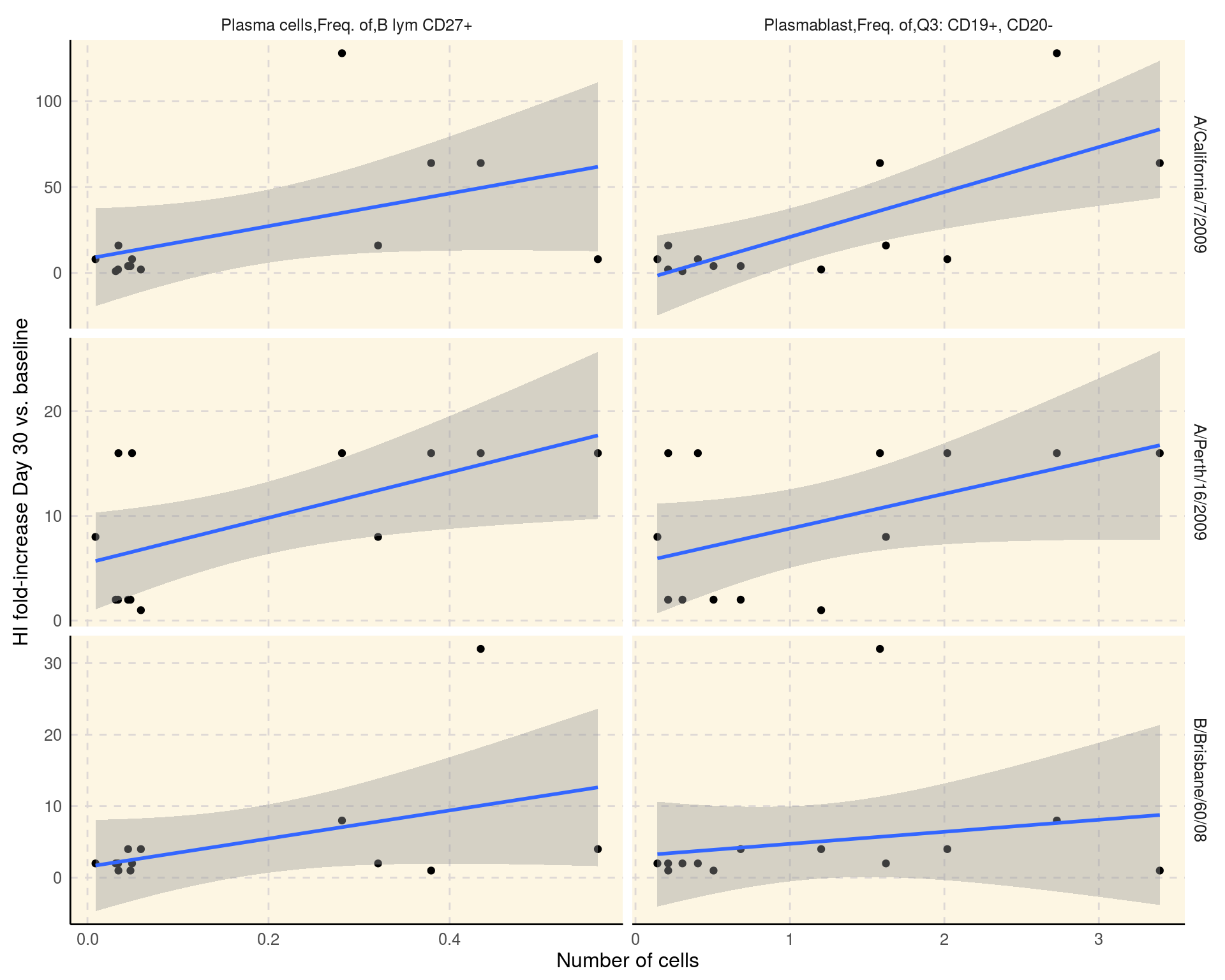### Correlations between hemagglutination inhibition (HI) and viral neutralization (VN) titers and plasmablast and plasma B cells among trivalent inactivated influenza vaccine (TIV) vaccinees.

This reports reproduces Figure 2 of Cao RG et al(2014) published as part of the original study.

``````library(ImmuneSpaceR)
library(data.table)
library(ggplot2)``````

#### Initialize the connection to SDY144 and get data

First we initialize the connection to the selected study using `CreateConnection`. Then we grab the datasets of interests with the `getDataset` method.

``````con <- CreateConnection("SDY144")
flow <- con\$getDataset("fcs_analyzed_result")
hai  <- con\$getDataset("hai")
vn   <- con\$getDataset("neut_ab_titer")``````

Then we select the cell populations and time points of intereset.

``````pb <- flow[population_name_reported %in% c("Plasma cells,Freq. of,B lym CD27+",
"Plasmablast,Freq. of,Q3: CD19+, CD20-")]
pb <- pb[, population_cell_number := as.numeric(population_cell_number)]
pb <- pb[study_time_collected == 7 & study_time_collected_unit == "Days"] #13 subjects
pb <- pb[, list(participant_id, population_cell_number, population_name_reported)]``````

We compute the HI and VN titer as the fold-increase between baseline and day 30.

``````# HAI
hai <- hai[,response:=value_reported/value_reported[study_time_collected==0],
by="virus,cohort,participant_id"][study_time_collected==30]
hai <- hai[, list(participant_id, virus, response)]
dat_hai <- merge(hai, pb, by = "participant_id", allow.cartesian = TRUE)
# VN
vn <- vn[, response:=value_reported/value_reported[study_time_collected==0],
by="virus,cohort,participant_id"][study_time_collected==30]
vn <- vn[, list(participant_id, virus, response)]
dat_vn <- merge(vn, pb, by = "participant_id", allow.cartesian = TRUE)``````

#### Plot using `ggplot2`

Figure 2 A: Correlation between the absolute number of plasmablasts and plasma Bcells 7 days after vaccination with and fold-increase of HI titers from baseline to day 30 after vaccination.

``````ggplot(dat_hai, aes(x = population_cell_number, y = response)) +
geom_point() + geom_smooth(method = "lm") +
facet_grid(virus~population_name_reported, scale = "free") +
xlab("Number of cells") + ylab("HI fold-increase Day 30 vs. baseline") + theme_IS()``````Figure 2 B: Correlation between the absolute number of plasmablasts and plasma Bcells 7 days after vaccination with and fold-increase of VN titers from baseline to day 30 after vaccination.

``````ggplot(dat_vn, aes(x = population_cell_number, y = response)) +
geom_point() + geom_smooth(method = "lm") +
facet_grid(virus~population_name_reported, scale = "free") +
xlab("Number of cells") + ylab("VN fold-increase Day 30 vs. baseline") + theme_IS()``````